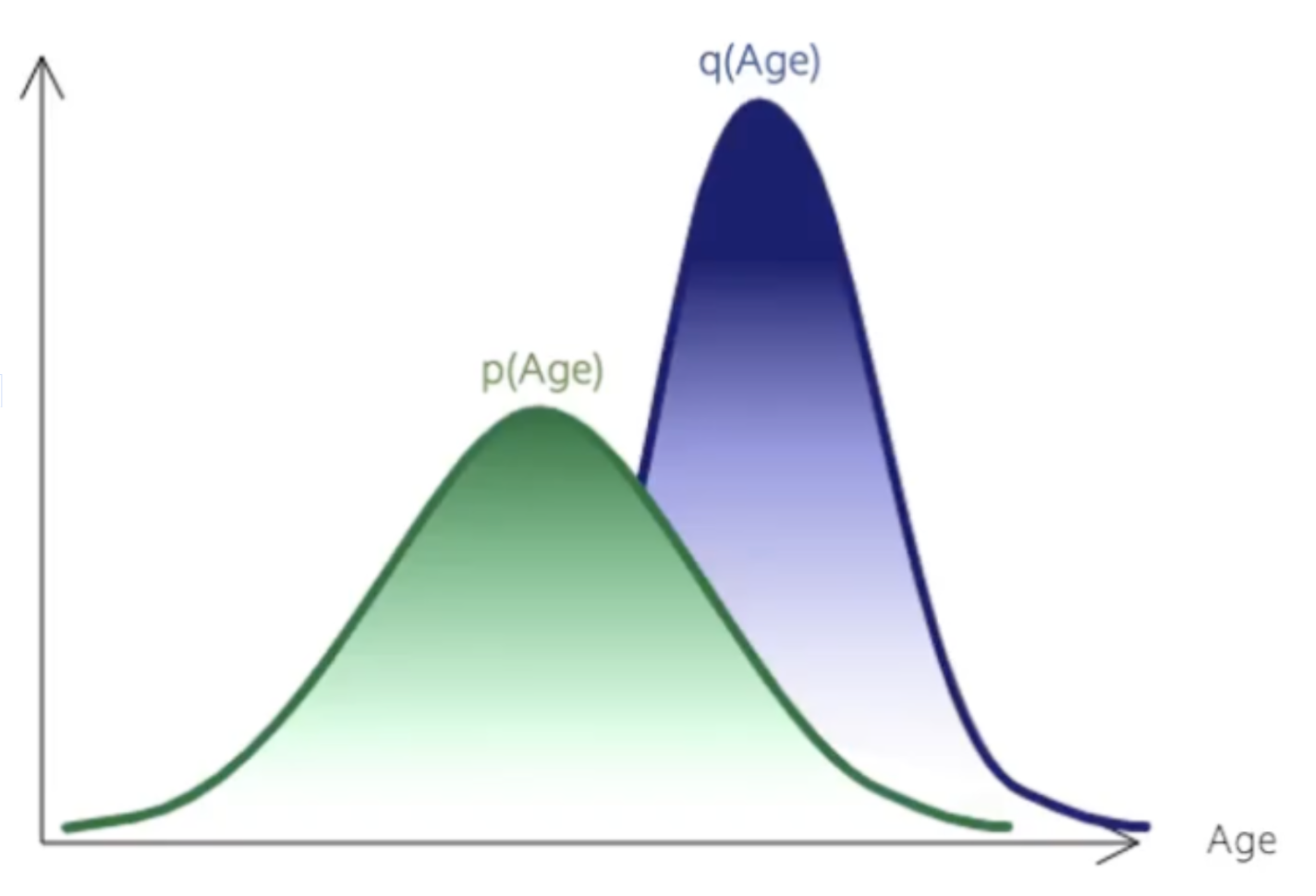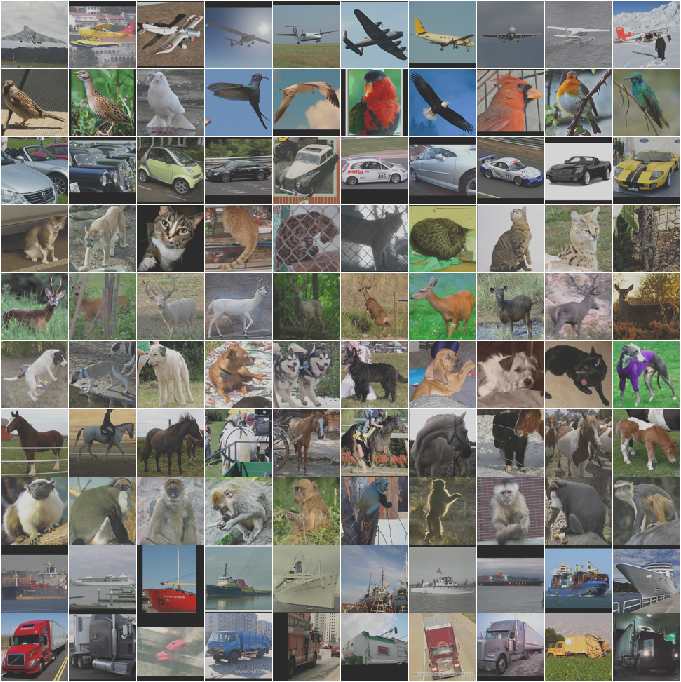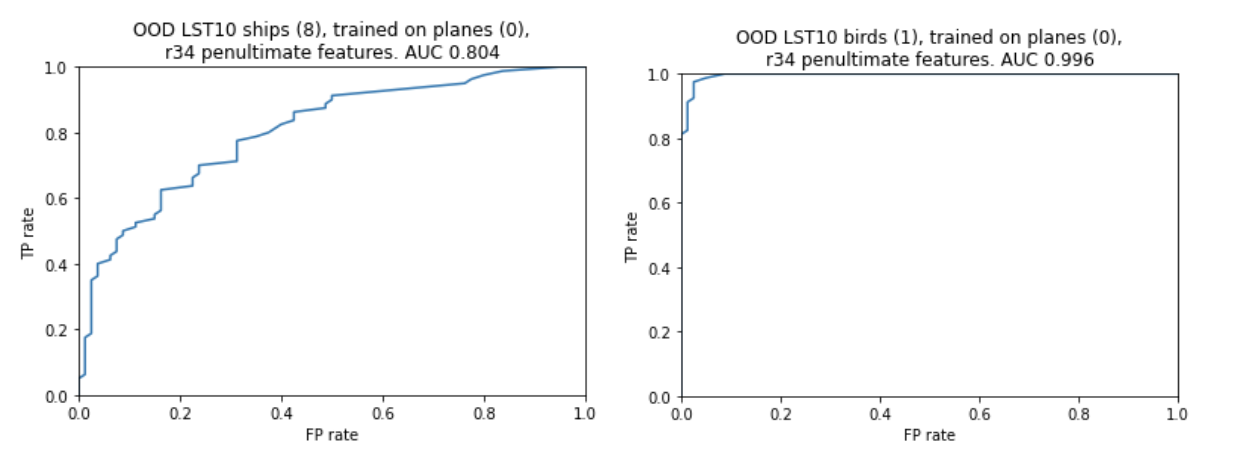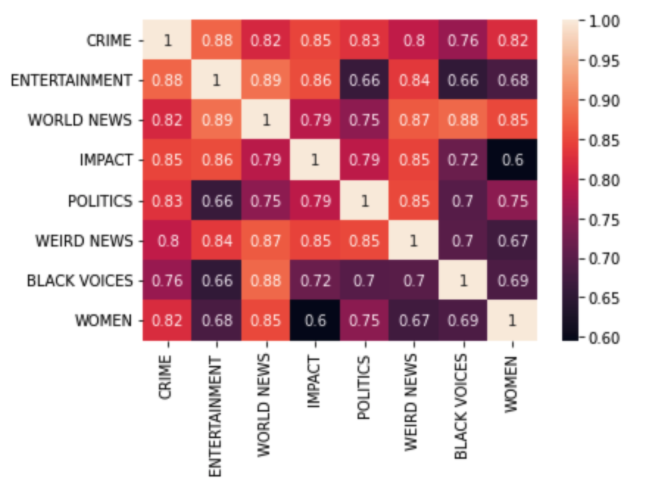Note: This is the second part of a series where we take a deeper dive into the question of data drift detection. If you haven't yet, check out the first part where we discussed data drift in the context of tabular data!

## Introduction

Data drift detection is a key component of a machine learning monitoring system. So far, we’ve discussed what data drift can look like in the context of tabular data, as well as some approaches to measuring drift. To recap, let’s revisit a simple example of data drift in a single feature:Comparing distributions. In this diagram, we examine a single input feature (Age) and look at the distribution of this variable at two time points. In the training data (green distribution) and in today’s production data (purple distribution).

In this case, the distribution of age in the training dataset is different from its distribution in a production environment. Over time, the performance of a model using age as an input feature can decay in response to the change in the environment the model is deployed in. There are a variety of metrics we can use for measuring the difference in these two distributions, but how do we measure drift without structured features? Systems trained on unstructured data, like text or images, face the same risks when deployed in production. However, detecting drift in these scenarios is more subtle, as we cannot use common divergence metrics on the raw data. In this post, we’ll walk through a general framework for data drift detection with unstructured data and we’ll highlight the two example use cases of NLP and computer vision.

Specifically, we aim to identify datapoints that are anomalous, or belonging to a distribution different than the training data. Formally, we would like to surface incoming datapoints that are likely to have been drawn from a distribution q(x) that is different from the training distribution p(x). We’ll rely on two common use cases to illustrate the out-of-distribution detection problem and evaluate our solution.

Our first example will be a computer vision use case, where the goal is to classify images based on the objects depicted in the image. For this setting, we used the STL-10 dataset from Stanford which provides high-resolution images from ten different possible classes including airplane, bird, dog, truck, and so on.Example images from the STL-10 dataset. We see images of cars, planes, trucks, dogs, etc. The STL-10 dataset contains 8,000 images.

Our second use case will be in NLP and we used a News Headline dataset which contains news headlines along with their respective topics such as crime, entertainment, world news, comedy, etc. Here, our objective is to classify headline text to the correct category.

## Algorithm/Approach

As with measuring multivariate drift in tabular data, the core motivation of the approach is to model the density, or distribution, of the reference dataset.

### Overview

There are several different approaches for finding anomalies in unstructured data. For any given approach, the three main aspects to determine anomalies in unseen data require:

1. Vector Representation: Convert the unstructured data to a vector embedding.
2. Density Model: Define a density model for the reference dataset.
3. Scoring: Create a method for scoring new datapoints against the reference density model.

In this section, we will discuss the variety of different techniques used for each of these three different components. Further, we will highlight example results with NLP and computer vision datasets.

### Vector Representation

We must convert our image or text data into a meaningful vector representation in order to understand the underlying distribution of the reference dataset. These vector representations are a type of feature extraction that can capture a useful representation of our unstructured data. Transfer learning is one approach for creating these representations by extracting embeddings of each image or text sequence from a large pre-trained model. These large-scale models are generally trained on millions of different datapoints and use state of the art architectures (CNN’s for image data or Transformers for text data) that can take unseen datapoints and produce a meaningful vector representation. For images, pre-trained models such as ResNet, VGG, or similar will be appropriate. For NLP data, we need to extract document embeddings and turn to pre-trained (or fine-tuned) Large Language Models.

While these are just a few examples of large-scale pre-trained models, there exist several others which are trained on different neural-network architectures and different datasets. This approach can be used with any type of vector embedding as long as it is meaningful for the context of your machine learning task.

### Density Model

Once we have meaningful vector abstractions for every point in our reference dataset, we must now create a density model that can model the underlying distribution. We can train a flexible density model to these embedding vectors. This could be accomplished with many possible techniques such as an auto-encoder, a VAE, a Normalizing Flow, a GAN, etc. In each case, this density model learns the structure and distribution of the reference set images or text (as represented in the embedding space).

As an example, auto-encoders are frequently used for unsupervised anomaly detection. Auto-encoders learn the latent representations of the reference set (consisting of vector embeddings) by encoding the vector to a lower dimensional vector and then decoding that representation back to its original dimension. We refer to the error measurement between the original input vector and the output vector as the reconstruction loss. Datapoints that are similar to points from the reference distribution will have a lower reconstruction error than points that are very different from the reference distribution. This property is useful for finding outliers as points that are outside the distribution of the reference set will have a high reconstruction error.

Taking a look at our news headline example, we can inspect the space learned by our auto-encoder. We first train the model on news headlines categorized as CRIME, which we treat as our in-distribution data. Below is a visualization of held-out crime headlines, as well as entertainment headlines.

### Scoring

Once we have trained our density model on our reference set, we must find a way to convert the reconstruction loss values from the model to actionable anomaly scores. Our approach is outlined below:

1. After training the model, we compute the reconstruction error of a holdout set (subset of the reference set) to use as a proxy distribution.
2. For every unseen datapoint, we compute the reconstruction error after being fed through our trained density model.
3. We find the percentile that our reconstruction error falls into relative to the reconstruction errors of the holdout set.

The motivation for our approach is twofold:

1. A lower reconstruction error means that the point is less likely to be anomalous (because the auto-encoder has seen many examples like it). Therefore, if an unseen datapoint yields a high reconstruction error (larger than anything from the holdout set), it is likely to be anomalous.
2. Because we rank in terms of percentiles, all our scores are normalized between 0 and 1. This makes it user-friendly and interpretable. Points that are close to 1 are more likely to be anomalous than points close to 0.

## Evaluation/Results

There are very few open-source datasets that have labeled data to measure anomaly detection for unstructured data types. Therefore, we constructed a few different test cases with our example datasets introduced earlier in this paper to measure the efficacy of our anomaly detection algorithm for unstructured data.

For each dataset (News Headlines and STL-10), we broke up our test cases as follows:

1. We segment our datapoints into in-distribution and out-of-distribution sets based on the labeled classes (e.g. all images as airplanes would be the reference set and all images as cars would be the out-of-distribution test set).
2. We furthermore segment the in-distribution dataset into 80% as the training set, and 20% as the holdout set. We use this to determine if our model would classify previously unseen datapoints as “anomalous” or “non-anomalous” based on the image object. For example, we would expect images from the in-class set should have low anomaly scores (near 0) and images from the out-of-class set should have high anomaly scores (near 1).
3. We run this test across different pairs of classes available in our dataset. We compute the AUC scores with relation to the “classification” of each datapoint as anomalous or non-anomalous (based on our scoring methodology).

We highlight two graphics below showcasing the results of our experiments.CV OOD: ROC Curves for STL-10 data where the in-distribution dataset is the class Planes, and the out-of-distribution class on the left is Ships and the out-of-distribution class on the left is Birds.

The figures above are showcasing the ROC curves for two specific experiments we ran using the STL-10 dataset. The graph on the left is measuring the AUC when the in-distribution dataset (non-anomalous) was taken from a set of ship images while the out-of-distribution dataset (anomalous) was taken from a set of plane images. Similarly, the graph on the right shows the ROC curve where the in-distribution dataset was taken from a set of bird images and the out-of-distribution dataset was taken from a set of plane images. We notice that for both experiments, the anomaly detector does a very good job (AUC scores of 0.804 and 0.996) of being able to differentiate between in-distribution and out-of-distribution datapoints.NLP OOD: News Headlines dataset divided by each category as the reference set. Compares cross-class anomaly accuracies (where another class is all “anomalous”).

The heatmap above is reporting the AUC scores for all possible pairwise experiments between possible classes in the news headline dataset (such as Crime, Entertainment, etc.). For any given cell in the heatmap, we are reporting the AUC score where the category on the x-axis is the in-distribution (non-anomalous) dataset while the category on the y-axis is the out-of-distribution (anomalous) dataset. We reported an average AUC score (across all crosswise pairs) to be 0.83, which is quite impressive given this task is difficult even for humans.

## Conclusion

This approach to out-of-distribution detection is especially powerful because it is completely unsupervised. In a production environment, we often don’t have prior knowledge of what kind of distribution shifts to expect or access to labeled data. Additionally, while we have considered two classification problems in this post, this technique can be applied to any type of machine learning task, as it only considers the input data and is therefore independent of the underlying ML task.

Detection of out-of-distribution samples is only the first step in maintaining a robust machine learning system. Monitor your ML model for drift with Arthur. At Arthur, we’re helping data scientists and machine learning engineers detect, understand, and respond to unforeseen production environments.